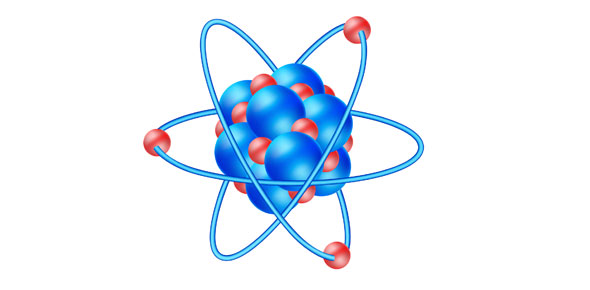# Atomic Structure And Kinetic Theory Of Matter

Approved & Edited by ProProfs Editorial Team
At ProProfs Quizzes, our dedicated in-house team of experts takes pride in their work. With a sharp eye for detail, they meticulously review each quiz. This ensures that every quiz, taken by over 100 million users, meets our standards of accuracy, clarity, and engagement.
| Written by Sstsci
S
Sstsci
Community Contributor
Quizzes Created: 8 | Total Attempts: 2,170
Questions: 10 | Attempts: 224SettingsThe objective of this quiz is to find out your pre-knowledge on Atomic Structure and Kinetic Theory of Matter
Before starting the quiz, key in your name, class and index no. In the NAME and ID fields.
Example: Name: Alpha Boon Chin Dee ID: S109_01

• 1.

### The atom is a basic unit of matter

• A.

True

• B.

False

A. True
Explanation
The statement is true because an atom is indeed considered the fundamental building block of matter. Atoms are the smallest units of an element that retain the chemical properties of that element. They consist of a nucleus, which contains protons and neutrons, and electrons that orbit the nucleus. All matter, whether it is solid, liquid, or gas, is composed of atoms. Therefore, it is correct to say that an atom is a basic unit of matter.

Rate this question:

• 2.

### What are sub-atomic atom?

• A.

Protons

• B.

Electrons

• C.

Neutrons

• D.

All of the above

D. All of the above
Explanation
The sub-atomic particles are the building blocks of an atom. Protons, electrons, and neutrons are all sub-atomic particles. Protons have a positive charge and are found in the nucleus of an atom. Electrons have a negative charge and orbit around the nucleus. Neutrons have no charge and are also found in the nucleus. Therefore, all of these particles are considered sub-atomic particles, making "All of the above" the correct answer.

Rate this question:

• 3.

### Which atomic particles will you find inside of the nucleus of an atom?

• A.

Protons + Electrons

• B.

Electrons + Neutrons

• C.

Protons + Neutrons

C. Protons + Neutrons
Explanation
Inside the nucleus of an atom, you will find protons and neutrons. Protons have a positive charge, while neutrons have no charge. Electrons, on the other hand, are found outside the nucleus in energy levels or orbitals. They have a negative charge and are involved in chemical reactions and bonding. Therefore, the correct answer is "Protons + Neutrons."

Rate this question:

• 4.

### Electrons carry _____ charge; protons carry ______ charge; neutrons carry ____ charge.

• A.

No; positive; negative

• B.

Negative; no; positive

• C.

Negative; positive; no

C. Negative; positive; no
Explanation
Electrons carry a negative charge because they have an excess of negatively charged particles. Protons carry a positive charge because they have an excess of positively charged particles. Neutrons, on the other hand, have no charge because they have an equal number of positively and negatively charged particles, resulting in a neutral charge.

Rate this question:

• 5.

### The relative mass of a neutron is the same as a proton

• A.

True

• B.

False

A. True
Explanation
The relative mass of a neutron is the same as a proton because both particles have a mass of approximately 1 atomic mass unit (amu). Neutrons and protons are both found in the nucleus of an atom and are collectively known as nucleons. While protons carry a positive charge and neutrons have no charge, their masses are very similar. This similarity in mass allows for the stability and structure of atomic nuclei.

Rate this question:

• 6.

### In a hydrogen gas molecule there more than 2 atoms of hydrogen

• A.

True

• B.

False

B. False
Explanation
The statement is false because a hydrogen gas molecule contains only 2 atoms of hydrogen.

Rate this question:

• 7.

### Calculate the relative molecular mass of one water molecule. (Given the atomic mass of oxygen is 16 and hydrogen is 1)

• A.

16

• B.

17

• C.

18

• D.

19

C. 18
Explanation
The relative molecular mass of one water molecule can be calculated by adding the atomic masses of its constituent atoms. In this case, there are two hydrogen atoms with an atomic mass of 1 each and one oxygen atom with an atomic mass of 16. Adding these together gives a total of 18, which is the relative molecular mass of one water molecule.

Rate this question:

• 8.

### According to the Kinetic Theory of Matter, gas molecules have the highest kinetic energy.

• A.

True

• B.

False

A. True
Explanation
According to the Kinetic Theory of Matter, gas molecules are in constant motion and have the highest kinetic energy compared to solids and liquids. This is because gas molecules have more freedom to move and are not held together by strong forces like in solids and liquids. Therefore, the statement "gas molecules have the highest kinetic energy" is true.

Rate this question:

• 9.

### According to Kinetic Theory of Matter, molecules in the solid state has the highest kinetic energy

• A.

True

• B.

False

B. False
Explanation
According to the Kinetic Theory of Matter, molecules in the solid state have the lowest kinetic energy compared to molecules in the liquid and gas states. In solids, the molecules are closely packed together and have limited movement, resulting in lower kinetic energy. In contrast, molecules in liquids and gases have more freedom to move and therefore higher kinetic energy. Therefore, the statement that molecules in the solid state have the highest kinetic energy is false.

Rate this question:

• 10.

### Which of the following transfer of heat processes explain the sea and land breeze?

• A.

Conduction

• B.

Convection

• C.Back to top